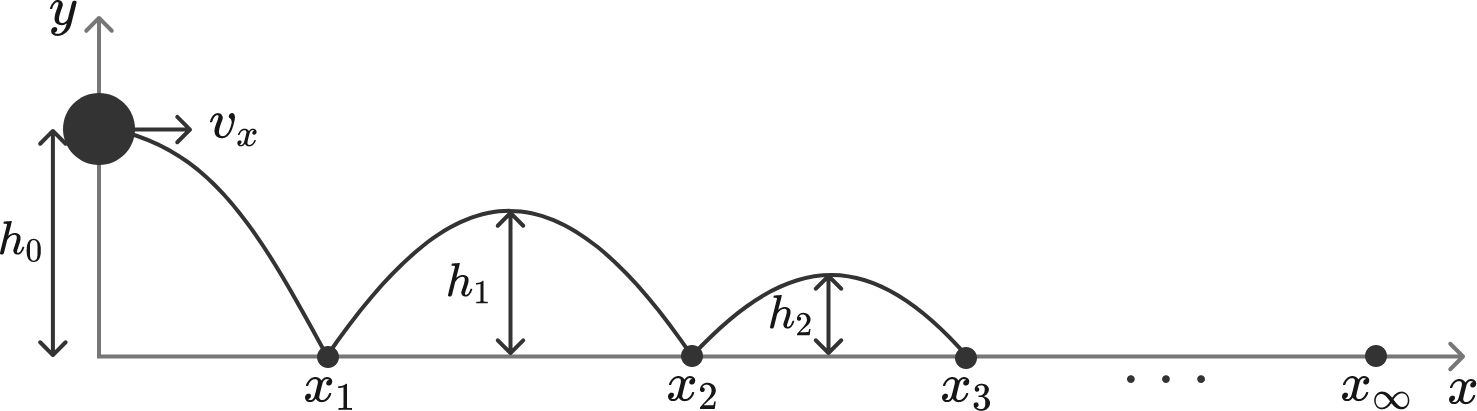# Bouncing ball

A ball thrown in the horizontal direction bounces off the ground, as illustrated in the diagram. Eventually, the ball stops bouncing and rolls on the ground. What is the total bounced distance $x_\infty,$ rounded to the nearest integer?


Details and Assumptions:

• The initial height of the ball is $h_0 = 0.8 \text{ m}.$
• The horizontal velocity of the ball is $v_x = 2.6 \text{ m/s}.$
• The gravitational acceleration is $g = 10 \text{ m/s}^2.$
• After each bounce, the ball loses 19% of its vertical kinetic energy $\frac{1}{2} m v_y^2$, whereas the horizontal motion is unaffected.×